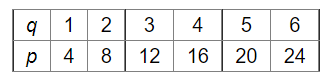# Plot a line graph for the variables p and q, where p is four times q, i.e., the equation is p = 4q.`
Question:

Plot a line graph for the variables p and q, where p is four times q, i.e., the equation is p = 4q.

Using the graph, find the value of (i) p, when = 6 and (ii) q, when p = 20.

Solution:

We have $p=4 q$

(i) When $q=6$

then $p=4 \times 4=16$

(ii) when $p=20$

then, $20=4 \mathrm{q}$

$5=\mathrm{q}$

And the graph of p = 4q is given by